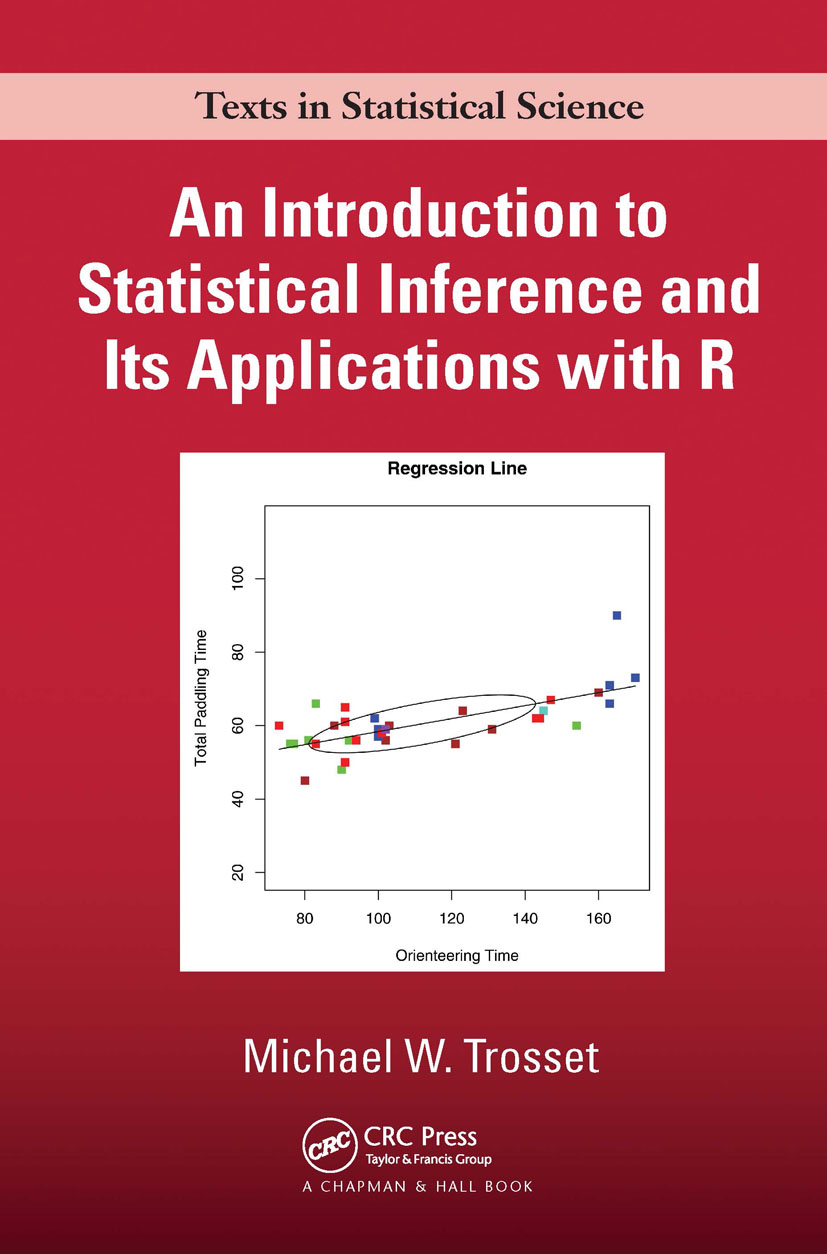An Introduction to Statistical Inference and Its Applications with R

1st Edition

Chapman and Hall/CRC

496 pages | 72 B/W Illus.

Purchasing Options:\$ = USD
Hardback: 9781584889472
pub: 2009-06-23
SAVE ~\$20.00
\$100.00
\$80.00
x
eBook (VitalSource) : 9780429148491
pub: 2009-06-23
from \$50.00

FREE Standard Shipping!

Description

Emphasizing concepts rather than recipes, An Introduction to Statistical Inference and Its Applications with R provides a clear exposition of the methods of statistical inference for students who are comfortable with mathematical notation. Numerous examples, case studies, and exercises are included. R is used to simplify computation, create figures, and draw pseudorandom samples—not to perform entire analyses.

After discussing the importance of chance in experimentation, the text develops basic tools of probability. The plug-in principle then provides a transition from populations to samples, motivating a variety of summary statistics and diagnostic techniques. The heart of the text is a careful exposition of point estimation, hypothesis testing, and confidence intervals. The author then explains procedures for 1- and 2-sample location problems, analysis of variance, goodness-of-fit, and correlation and regression. He concludes by discussing the role of simulation in modern statistical inference.

Focusing on the assumptions that underlie popular statistical methods, this textbook explains how and why these methods are used to analyze experimental data.

Experiments

Examples

Randomization

The Importance of Probability

Games of Chance

Mathematical Preliminaries

Sets

Counting

Functions

Limits

Probability

Interpretations of Probability

Axioms of Probability

Finite Sample Spaces

Conditional Probability

Random Variables

Case Study: Padrolling in Milton Murayama’s All I asking for is my body

Discrete Random Variables

Basic Concepts

Examples

Expectation

Binomial Distributions

Continuous Random Variables

A Motivating Example

Basic Concepts

Elementary Examples

Normal Distributions

Normal Sampling Distributions

Quantifying Population Attributes

Symmetry

Quantiles

The Method of Least Squares

Data

The Plug-In Principle

Plug-In Estimates of Mean and Variance

Plug-In Estimates of Quantiles

Kernel Density Estimates

Case Study: Are Forearm Lengths Normally Distributed?

Transformations

Lots of Data

Averaging Decreases Variation

The Weak Law of Large Numbers

The Central Limit Theorem

Inference

A Motivating Example

Point Estimation

Heuristics of Hypothesis Testing

Testing Hypotheses about a Population Mean

Set Estimation

1-Sample Location Problems

The Normal 1-Sample Location Problem

The General 1-Sample Location Problem

The Symmetric 1-Sample Location Problem

Case Study: Deficit Unawareness in Alzheimer’s Disease

2-Sample Location Problems

The Normal 2-Sample Location Problem

The Case of a General Shift Family

Case Study: Etruscan versus Italian Head Breadth

The Analysis of Variance

The Fundamental Null Hypothesis

Testing the Fundamental Null Hypothesis

Planned Comparisons

Post Hoc Comparisons

Case Study: Treatments of Anorexia

Goodness-of-Fit

Partitions

Test Statistics

Testing Independence

Association

Bivariate Distributions

Normal Random Variables

Monotonic Association

Explaining Association

Case Study: Anorexia Treatments Revisited

Simple Linear Regression

The Regression Line

The Method of Least Squares

Computation

The Simple Linear Regression Model

Assessing Linearity

Case Study: Are Thick Books More Valuable?

Simulation-Based Inference

Termite Foraging Revisited

The Bootstrap

Case Study: Adventure Racing

R: A Statistical Programming Language

Introduction

Using R

Functions That Accompany This Book

Index

Exercises appear at the end of each chapter.